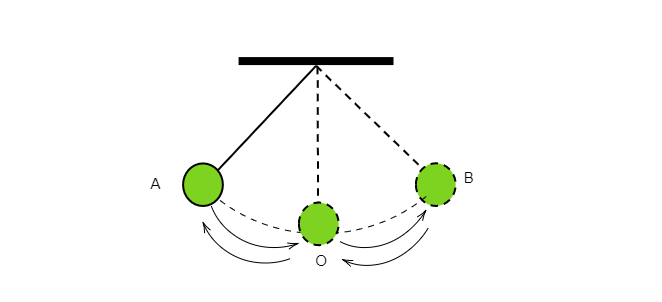# A simple pendulum is oscillating between two points A and B as shown in the figure. Is the motion of the bob uniform or non-uniform?"

The speed of the bob in a simple pendulum changes every time from A to B. So, its motion can't be a uniform motion. Therefore, the motion of the bob in a simple pendulum is a non-uniform motion.